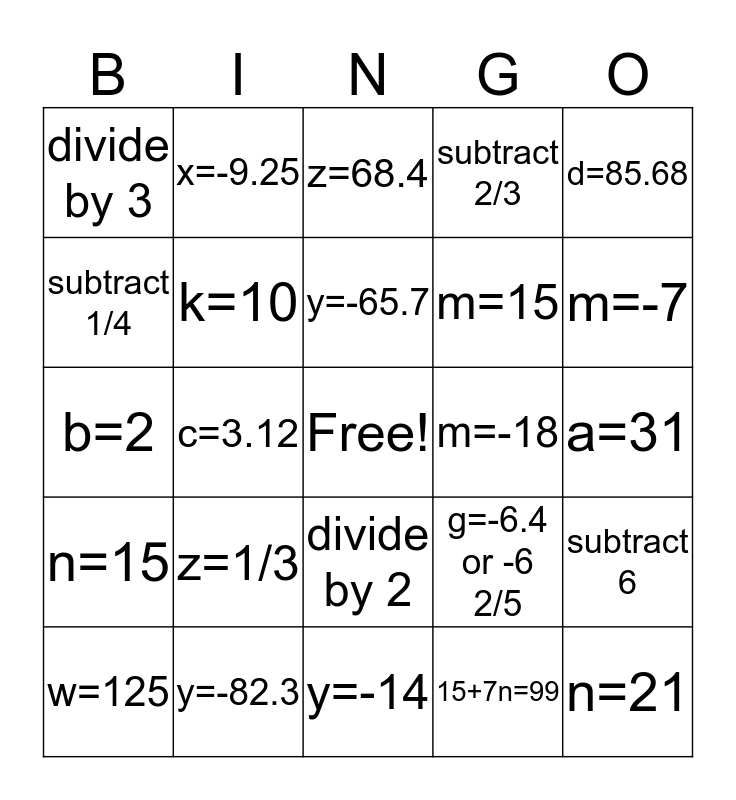# Algebraic EquationsThis bingo card has a free space and 24 words: z=1/3, y=-82.3, y=-65.7, w=125, d=85.68, divide by 3, subtract 1/4, x=-9.25, m=-18, divide by 2, m=15, b=2, g=-6.4 or -6 2/5, subtract 6, k=10, 15+7n=99, a=31, z=68.4, m=-7, c=3.12, n=21, n=15, subtract 2/3 and y=-14.

## Play Online